User:IssaRice/Minus notation in game theory

In game theory,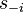$s_{-i}$ is a shorthand for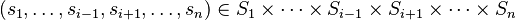$(s_1,\ldots,s_{i-1},s_{i+1},\ldots,s_n) \in S_1 \times \cdots \times S_{i-1} \times S_{i+1} \times \cdots \times S_n$. In other words,$s_{-i}$ is a tuple of strategies of all the players other than player$i$.
One then writes things like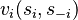$v_i(s_i, s_{-i})$ for the payoff of the strategy profile where each player$i$ chooses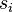$s_i$.
But to my mind, there is a type error here, because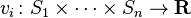$v_i \colon S_1 \times \cdots \times S_n \to \mathbf R$ is supposed to take the strategies in order, whereas in the previous paragraph, for each player, their own strategy appears first. To give an example, in the case of$n=2$, each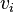$v_i$ should take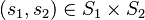$(s_1,s_2) \in S_1\times S_2$, but the notation$(s_i, s_{-i})$ for player 2 is$(s_2,s_1)$, which is reversed. Type error!
I think usually this is not a problem, because the reader can mentally put the$s_i$ in the right spot, or one can assume that no two strategies (even across different players) are the same (e.g. the strategies can be "marked" with numbers to be distinguishable), so that one can pass a set of strategies like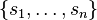$\{s_1, \ldots ,s_n\}$ and there would be no confusion even if the ordering is different.
• One can change each$v_i$ to have domain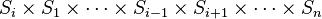$S_i\times S_1 \times \cdots \times S_{i-1} \times S_{i+1} \times \cdots \times S_n$. This is sort of inelegant because now one cannot pass$s \in S_1\times \cdots \times S_n$ to an arbitrary$v_i$. (One can only pass it to$v_1$.)
• One can define a function$g_i$ for each player that is supposed to "put$s_i$ back in the right spot". Formally,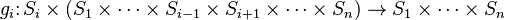$g_i\colon S_i \times (S_1 \times \cdots \times S_{i-1} \times S_{i+1} \times \cdots \times S_n) \to S_1 \times \cdots \times S_n$ is defined by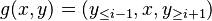$g(x,y) = (y_{\leq i-1}, x, y_{\geq i+1})$. Now one can write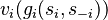$v_i(g_i(s_i, s_{-i}))$ (if the$i$th strategy needs to be put in the correct place) and$v_i(s)$ (if the strategies are already in order). Note that the altered$v_i$ suggested in the previous bullet is, in this notation,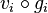$v_i \circ g_i$.